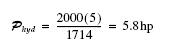﻿ Basic Concept of Hydraulic Cylinder - Hydraulic Schematic Troubleshooting

## Basic Concept of Hydraulic Cylinder

Suppose a flow of fluid is delivered to a hydraulic cylinder, causing it to extend. The cylinder has a cross-sectional area A and delivers a force F while moving a distance x (Fig. 1.2). The distance moved is related to the fluid volume delivered to the cylinder.

x = V/A                    (1.5)

where
x = distance (in)
V = volume (in3 )
A = area (in2)

The force is related to the pressure developed at the cap end.

F = PA                      (1.6)

where
F = force (lbf)
P = pressure (lbf/in2)
A = area (in2)

(Throughout this text, pressure in lbf/in2 is expressed as psi.)
Work done is given by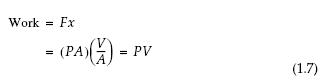Power is work per unit time,

Power = PV/t                   (1.8)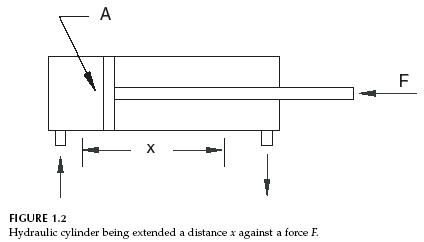Flow is defined as volume per unit time, Q = V/t ; therefore,

Power = PQ                             (1.9)

Mechanical power is the product of T and N and hydraulic power is the product of P and Q.

The relationship in Eq. (1.9) is the fundamental concept for our study of fluid power. The units used for pressure are typically lbf/in2, or psi, and the units for flow are gal/min, or GPM. To obtain hydraulic power with units of lbf-ft/min, the following conversions are needed.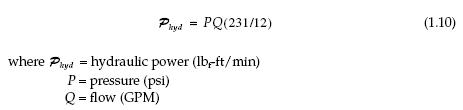To obtain hydraulic horsepower,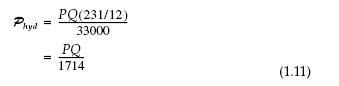It is useful to memorize this formula, as it will give a quick frame of reference when beginning a design. For example, if a pump delivers 5 GPM at 2,000 psi pressure drop, how much power is required?